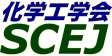## \$B9V1i%W%m%0%i%`!J2q>l!&F|DxJL!K(B

### P\$B2q>l(B \$BBh(B2\$BF|(B

\$B:G=*99?7F|;~!'(B2007-03-18 23:33:23

2006\$BG/(B12\$B7n(B2\$BF|!A(B24\$BF|\$K7G:\\$5\$l\$F\$\$\$?2>%W%m%0%i%`\$H\$O2q>lL>\$,JQ99\$5\$l\$F\$\$\$^\$9\$N\$G\$4Cm0U\$/\$@\$5\$\$!#(B

\$B9V1i(B
\$B;~9o(B
\$B9V1i(B
\$BHV9f(B
\$B9V1iBjL\!?H/I=\$B%-!<%o!<%I(B\$BJ,N`(B
\$BHV9f(B
\$B\$BHV9f(B
\$B4D6-(B \$B!]%(%M%k%.!<\$H:F;q8;2=!](B
(9:00\$B!A(B10:00)\$B!!(B(\$B:BD9(B \$B8eF#(B \$B85?.(B)
9:00\$B!A(B 9:20P201\$B0!NW3&?e=hM}\$K\$h\$k%*%+%i\$N;q8;2=(B
(\$B:eI\Bg1!9)(B) (\$B3X(B)\$BCg0f(B \$B9/Fs(B\$B!&(B\$B!{(B(\$B@5(B)\$B5HED(B \$B90G7(B
subcritical water
okara
zero emission
13-a752
9:20\$B!A(B 9:40P202\$B0!NW3&?e=hM}\$K\$h\$k%*%+%i\$N2C?eJ,2rH?1~F0NO3X(B
(\$B:eI\Bg1!9)(B) \$B!{(B(\$B3X(B)\$BCg0f(B \$B9/Fs(B\$B!&(B(\$B@5(B)\$B5HED(B \$B90G7(B\$B!&(B(\$B@5(B)\$BCfB<(B \$B=(H~(B
subcritical water
okara
kinetics
13-a758
9:40\$B!A(B 10:00P203\$B2C059b29=hM}\$K\$h\$kLZ
(\$BBgJ,Bg9)(B) \$B!{(B(\$B3X(B)\$B0f>e(B \$B5.8x(B\$B!&(B\$BJ?2,(B \$BFX(B\$B!&(B(\$B@5(B)\$BT"J,(B \$B=\$;0(B\$B!&(B(\$B@5(B)\$BDL:e(B \$B1I0l(B\$B!&(B(\$B@5(B)\$BJ?ED(B \$B@?(B\$B!&(B(\$BBgJ,Bg(B) (\$B@5(B)\$B1)Ln(B \$BCi(B
combined treatment of high pressure and heat
waste wood
recycled board
13-e827
(10:00\$B!A(B11:00)\$B!!(B(\$B:BD9(B \$BJ?ED(B \$B@?(B)
10:00\$B!A(B 10:20P204\$B0!NW3&?e=hM}\$K\$h\$k%\%?%s%&%-%/%5\$N;q8;!&%(%M%k%.!<2=(B
(\$B:eI\Bg1!9)(B) \$B!{(B(\$B@5(B)\$B9uLZ(B \$B@,0lO/(B\$B!&(B(\$B@5(B)\$B5HED(B \$B90G7(B
sub-critical water
waste treatment
water plants
13-a793
10:20\$B!A(B 10:40P205\$BA!0]6/2=%W%i%9%A%C%/\$N0!NW3&=hM}\$K\$h\$k86NA@.J,\$N2s<}(B
(\$B7'Bg9)(B) \$B!{7,ED(B \$BM}9>(B\$B!&(B\$B5HED(B \$B6G90(B\$B!&(B(\$B7'Bg1!<+(B) (\$B3X(B)\$B4dC+(B \$BCR;R(B\$B!&(B(\$B7'Bg9)(B) (\$B@5(B)\$B:4!9LZ(B \$BK~(B\$B!&(B(\$B7'Bg1!<+(B) (\$B@5(B)\$B8eF#(B \$B85?.(B\$B!&(B(\$BF|N)2=@.9)6H(B) \$B!;J(B
Fiber Reinforced Plastic(FRP)
Recycling
Sub-critical
13-e613
10:40\$B!A(B 11:00P206\$B?eG.H?1~\$rMQ\$\$\$?%]%jF};@%1%_%+%k%j%5%\$%/%k\$K\$*\$1\$k(BLCI\$BI>2A(B
(\$BK-665;Bg(B) \$B!{(B(\$B3X(B)\$B?7J](B \$BC#M:(B\$B!&(B(\$B@5(B)\$BG.ED(B \$BMN0l(B\$B!&(B(\$B@5(B)\$BBgLg(B \$BM5G7(B\$B!&(B(\$B@5(B)\$BF#9>(B \$B9,0l(B
poly lactic acid
chemical recycle
life cycle inventory
13-e612
(11:00\$B!A(B12:00)\$B!!(B(\$B:BD9(B \$BF#9>(B \$B9,0l(B)
11:00\$B!A(B 11:20P207\$B%]%jF};@%+%C%W\$N%1%_%+%k%j%5%\$%/%k\$H;v6H@-8&5f(B
(\$B6e9)Bg1!@8BN9)(B) \$B!{(B(\$B@5(B)\$BGr0f(B \$B5A?M(B\$B!&(B(\$B@5(B)\$BOF:d(B \$B9A(B\$B!&(B(\$B6&(B)\$BImLZ(B \$B5.9T(B\$B!&(B\$B1JED(B \$B9@0l(B\$B!&(B(\$BIpB"Ln2=3X(B) \$BC+8}(B \$B@5L@(B\$B!&(B\$B;01:(B \$B=E?.(B\$B!&(B(\$B6a5&BgJ,9)8&(B) (\$B6&(B)\$B@>ED(B \$B<#CK(B
PLA
Chemical Recycle
Biomass Plastic
13-e361
11:20\$B!A(B 11:40P208Comparative Study of Biodiesel Production by Alkaline Transesterification from Low-valued Feed
(TIT) \$B!{(B(\$B3X(B)Sinthupinyo Patima\$B!&(B(\$B@5(B)Ryuichi Egashira
biodiesel yield
feed oil comparison
pretreatment
13-i501
11:40\$B!A(B 12:00P209\$B%P%\$%*%G%#!<%<%kG3NA9g@.\$N\$?\$a\$N8GBN1v4p?(G^\$N3+H/8&5f(B
(\$B0&I2BgG@(B) \$B!{(B(\$B@5(B)\$B@nEh(B \$BJ8?M(B\$B!&(B(\$B3X(B)\$B>>86(B \$B^+(B\$B!&(B\$BK\ED(B \$B9n5W(B
biodiesel fuel
heterogeneous
base catalysis
13-e36

\$B9V1i%W%m%0%i%`(B
\$B2=3X9)3X2q(B \$BBh(B72\$BG/2q(B

(C) 2007 (\$B
Most recent update: 2007-03-18 23:33:23
For more information contact \$B2=3X9)3X2q4X@>;YIt(B \$BBh(B72\$BG/2q(B \$BLd\$\$9g\$;78(B
E-mail: inquiry-72awww2.scej.org
This page was generated byeasp 2.22; proghtml 2.20b (C)1999-2006 kawase